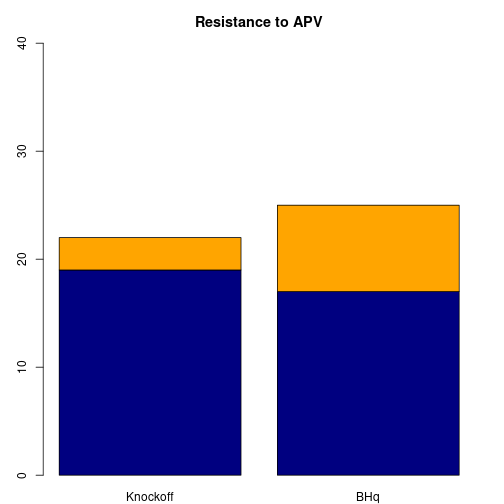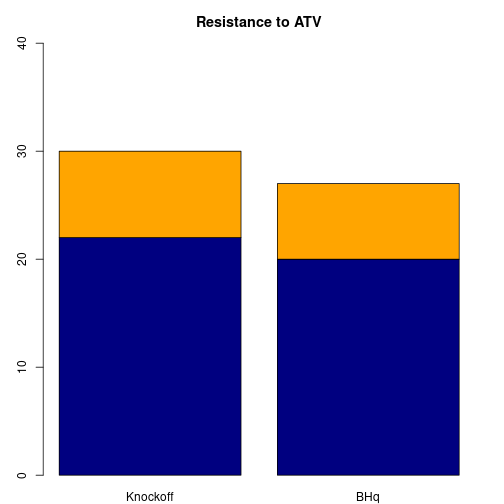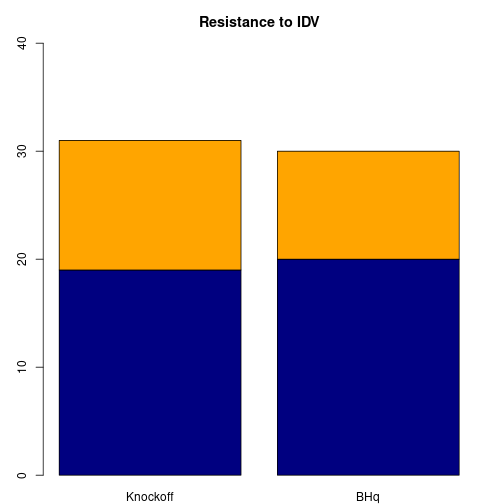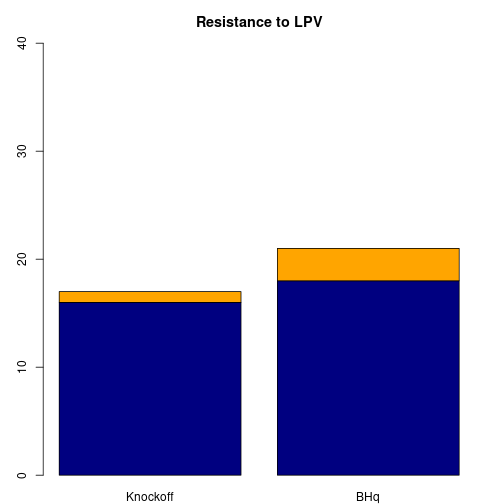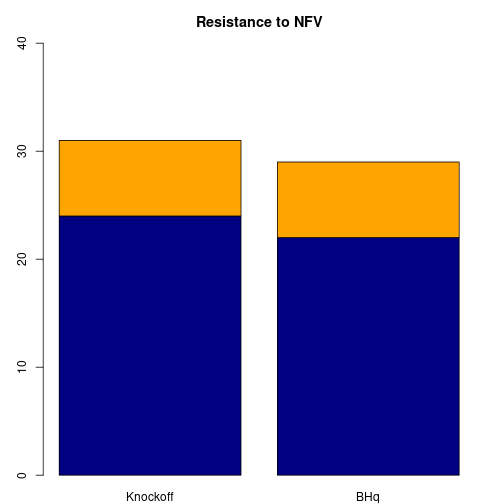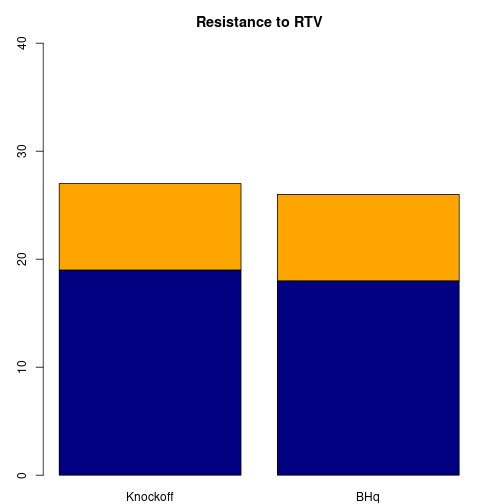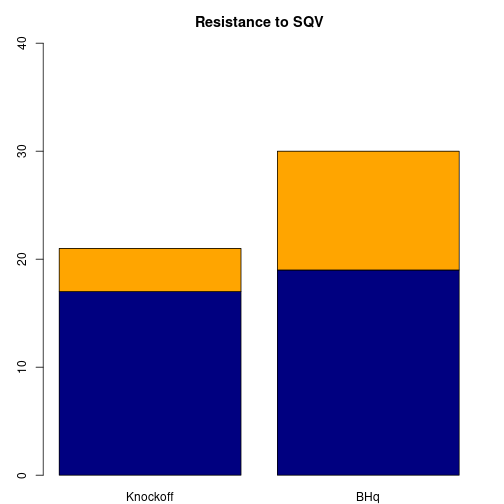In this vignette, we illustrate the analysis of a real (non-simulated) data set, including all the pre-processing steps. We hope this example will guide the user in their own applications of the knockoff filter. Throughout this example we will apply the knockoff filter in the Fixed-X scenario.

The scientific goal is to determine which mutations of the Human Immunodeficiency Virus Type 1 (HIV-1) are associated with drug resistance. The data set, publicly available from the Stanford HIV Drug Resistance Database, was originally analyzed in [@rhee2006]. The analysis described here is exactly that carried out for the knockoff filter paper [@barber2014].

# Preparing the data

The data set consists of measurements for three classes of drugs: protease inhibitors (PIs), nucleoside reverse transcriptase (RT) inhibitors (NRTIs), and nonnucleoside RT inhibitors (NNRTIs). Protease and reverse transcriptase are two enzymes in HIV-1 that are crucial to the function of the virus. This data set seeks associations between mutations in the HIV-1 protease and drug resistance to different PI type drugs, and between mutations in the HIV-1 reverse transcriptase and drug resistance to different NRTI and NNRTI type drugs.

In order to evaluate our results, we compare with the treatment-selected mutation panels created by [@rhee2005]. These panels give lists of HIV-1 mutations appearing more frequently in patients who have previously been treated with PI, NRTI, or NNRTI type drugs, than in patients with no previous exposure to that drug type. Increased frequency of a mutation among patients treated with a certain drug type implies that the mutation confers resistance to that drug type.

In this vignette, we will confine our attention to the PI drugs.

drug_class = 'PI' # Possible drug types are 'PI', 'NRTI', and 'NNRTI'.


## Fetching and cleaning the data

base_url = 'http://hivdb.stanford.edu/pages/published_analysis/genophenoPNAS2006'
gene_url = paste(base_url, 'DATA', paste0(drug_class, '_DATA.txt'), sep='/')
tsm_url = paste(base_url, 'MUTATIONLISTS', 'NP_TSM', drug_class, sep='/')

gene_df = read.delim(gene_url, na.string = c('NA', ''), stringsAsFactors = FALSE)
names(tsm_df) = c('Position', 'Mutations')


A small sample of the data is shown below.

APV ATV IDV LPV NFV RTV SQV P1 P2 P3 P4 P5 P6 P7 P8 P9 P10
2.3 NA 32.7 NA 23.4 51.6 37.8 - - - - - - - - - I
76.0 NA 131.0 200.0 50.0 200.0 156.0 - - - - - - - - - F
2.8 NA 12.0 NA 100.0 41.0 145.6 - - - - - - - - - -
6.5 9.2 2.1 5.3 5.0 36.0 13.0 - - - - - - - - - I
8.3 NA 100.0 NA 161.1 170.2 100.0 - - - - - - - - - I
82.0 75.0 400.0 400.0 91.0 400.0 400.0 - - - - - - - - - I
Position Mutations
10 F R
11 I
20 I T V
23 I
24 I
30 N

The gene data table has some rows with error flags or nonstandard mutation codes. For simplicity, we remove all such rows.

# Returns rows for which every column matches the given regular expression.
grepl_rows <- function(pattern, df) {
cell_matches = apply(df, c(1,2), function(x) grepl(pattern, x))
apply(cell_matches, 1, all)
}

pos_start = which(names(gene_df) == 'P1')
pos_cols = seq.int(pos_start, ncol(gene_df))
valid_rows = grepl_rows('^(\\.|-|[A-Zid]+)$', gene_df[,pos_cols]) gene_df = gene_df[valid_rows,]  ## Preparing the design matrix We now construct the design matrix $$X$$ and matrix of response vectors $$Y$$. The features (columns of $$X$$) are given by mutation/position pairs. Define $X_{i,j} = \text{1 if the ith patient has the jth mutation/position pair and 0 otherwise} \ Y_{i,j} = \text{resistance of patient i to drug j}.$ For example, in the sample for PI type drugs, three different mutations (A, C, and D) are observed at position 63 in the protease, and so three columns of $$X$$ (named P63.A, P63.C, and P63.D) indicate the presence or absence of each mutation at this position. # Flatten a matrix to a vector with names from concatenating row/column names. flatten_matrix <- function(M, sep='.') { x <- c(M) names(x) <- c(outer(rownames(M), colnames(M), function(...) paste(..., sep=sep))) x } # Construct preliminary design matrix. muts = c(LETTERS, 'i', 'd') X = outer(muts, as.matrix(gene_df[,pos_cols]), Vectorize(grepl)) X = aperm(X, c(2,3,1)) dimnames(X)[] <- muts X = t(apply(X, 1, flatten_matrix)) mode(X) <- 'numeric' # Remove any mutation/position pairs that never appear in the data. X = X[,colSums(X) != 0] # Extract response matrix. Y = gene_df[,4:(pos_start-1)]  An excerpt of the design matrix is shown below. By construction, every column contains at least one 1, but the matrix is still quite sparse with the relative frequency of 1's being about 0.025. P4.A P12.A P13.A P16.A P20.A P22.A P28.A P37.A P51.A P54.A 0 0 0 0 0 0 0 0 0 0 0 0 0 1 0 0 0 0 0 0 0 0 0 0 0 0 0 0 0 0 0 0 0 0 0 0 0 0 0 0 0 0 0 0 0 0 0 0 0 0 0 0 0 0 0 0 0 0 0 0 0 0 0 0 0 0 0 0 0 0 0 0 0 0 0 0 0 1 0 0 0 0 0 1 0 0 0 0 0 0 0 0 0 0 0 0 0 0 0 0 The response matrix looks like: APV ATV IDV LPV NFV RTV SQV 2.3 NA 32.7 NA 23.4 51.6 37.8 76.0 NA 131.0 200.0 50.0 200.0 156.0 2.8 NA 12.0 NA 100.0 41.0 145.6 6.5 9.2 2.1 5.3 5.0 36.0 13.0 8.3 NA 100.0 NA 161.1 170.2 100.0 82.0 75.0 400.0 400.0 91.0 400.0 400.0 Notice that there are some missing values. ## Preparing the response vector The knockoff filter is designed to control the FDR under Gaussian noise. A quick inspection of the response vector shows that it is highly non-Gaussian. hist(Y[,1], breaks='FD')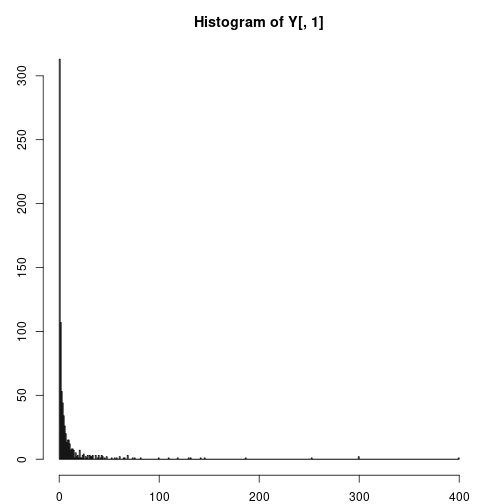A log-transform seems to help considerably, so we will use the log-transformed drug resistancement measurements below. hist(log(Y[,1]), breaks='FD')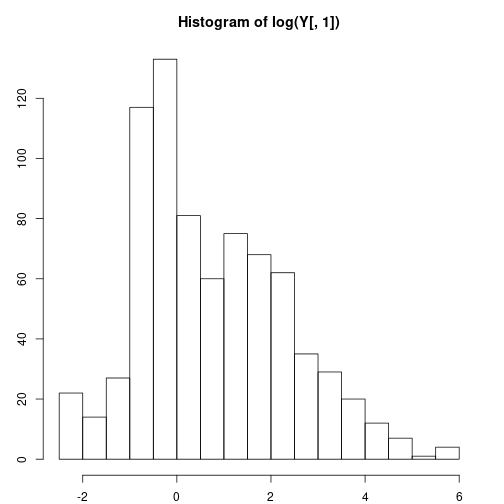# Running the knockoff filter We now run the knockoff filter on each drug separately. We also run the Benjamini-Hochberg (BHq) procedure for later comparison. Before running either selection procedure, we perform some final pre-processing steps. We remove rows with missing values (which vary from drug to drug) and we then further reduce the design matrix by removing predictor columns for mutations that do not appear at least three times in the sample. Finally, for identifiability, we remove any columns that are duplicates (i.e. two mutations that appear only in tandem, and therefore we cannot distinguish between their effects on the response). library(knockoff) knockoff_and_bhq <- function (X, y, q) { # Log-transform the drug resistance measurements. y = log(y) # Remove patients with missing measurements. missing = is.na(y) y = y[!missing] X = X[!missing,] # Remove predictors that appear less than 3 times. X = X[,colSums(X) >= 3] # Remove duplicate predictors. X = X[,colSums(abs(cor(X)-1) < 1e-4) == 1] # Run the knockoff filter. knock.gen = function(x) create.fixed(x, method='equi') result = knockoff.filter(X, y, fdr=fdr, knockoffs=knock.gen, statistic=stat.glmnet_lambdasmax) knockoff_selected = names(result$selected)

# Run BHq.
p = ncol(X)
lm.fit = lm(y ~ X - 1) # no intercept
p.values = coef(summary(lm.fit))[,4]
cutoff = max(c(0, which(sort(p.values) <= fdr * (1:p) / p)))
bhq_selected = names(which(p.values <= fdr * cutoff / p))

list(Knockoff = knockoff_selected, BHq = bhq_selected)
}

fdr = 0.20
results = lapply(Y, function(y) knockoff_and_bhq(X, y, fdr))


For example, here are the selected variables associated with the first drug:

print(results)

## $APV ##$APV$Knockoff ##  "P82.A" "P84.A" "P84.C" "P58.E" "P10.F" "P33.F" "P82.F" "P10.I" "P11.I" ##  "P24.I" "P32.I" "P46.I" "P46.L" "P50.L" "P54.L" "P48.M" "P54.M" "P90.M" ##  "P63.P" "P88.S" "P91.S" "P20.T" "P43.T" "P54.T" "P10.V" "P22.V" "P47.V" ##  "P48.V" "P50.V" "P54.V" "P71.V" "P76.V" "P84.V" ## ##$APV$BHq ##  "XP12.A" "XP84.A" "XP84.C" "XP58.E" "XP10.F" "XP33.F" "XP82.F" "XP10.I" ##  "XP24.I" "XP32.I" "XP46.I" "XP77.I" "XP82.I" "XP10.L" "XP46.L" "XP50.L" ##  "XP54.L" "XP48.M" "XP54.M" "XP90.M" "XP37.N" "XP69.Q" "XP43.R" "XP63.S" ##  "XP88.S" "XP91.S" "XP20.T" "XP43.T" "XP54.T" "XP82.T" "XP10.V" "XP47.V" ##  "XP50.V" "XP54.V" "XP64.V" "XP76.V" "XP84.V" "XP37.Y" "XP14.Z"  # Evaluating the results In this case, we are fortunate enough to have a “ground truth” obtained by another experiment. Using this, we compare the results from the knockoff and BHq procedures. Note that we compare only the position of the mutations, not the mutation type. This is because it is known that multiple mutations at the same protease or RT position can often be associated with related drug-resistance outcomes. get_position <- function(x) sapply(regmatches(x, regexpr('[[:digit:]]+', x)), as.numeric) comparisons <- lapply(results, function(drug) { lapply(drug, function(selected) { positions = unique(get_position(selected)) # remove possible duplicates discoveries = length(positions) false_discoveries = length(setdiff(positions, tsm_df$Position))
list(true_discoveries = discoveries - false_discoveries,
false_discoveries = false_discoveries,
fdp = false_discoveries / max(1, discoveries))
})
})


For example, here are the discoveries made by each procedure on the first drug.

print(comparisons)

## $APV ##$APV$Knockoff ##$APV$Knockoff$true_discoveries
##  19
##
## $APV$Knockoff$false_discoveries ##  3 ## ##$APV$Knockoff$fdp
##  0.1363636
##
##
## $APV$BHq
## $APV$BHq$true_discoveries ##  17 ## ##$APV$BHq$false_discoveries
##  8
##
## $APV$BHq\$fdp
##  0.32


## Visualization

Let's compare the discoveries graphically.

for (drug in names(comparisons)) {
plot_data = do.call(cbind, comparisons[[drug]])
plot_data = plot_data[c('true_discoveries','false_discoveries'),]
barplot(as.matrix(plot_data), main = paste('Resistance to', drug),
col = c('navy','orange'), ylim = c(0,40))
}